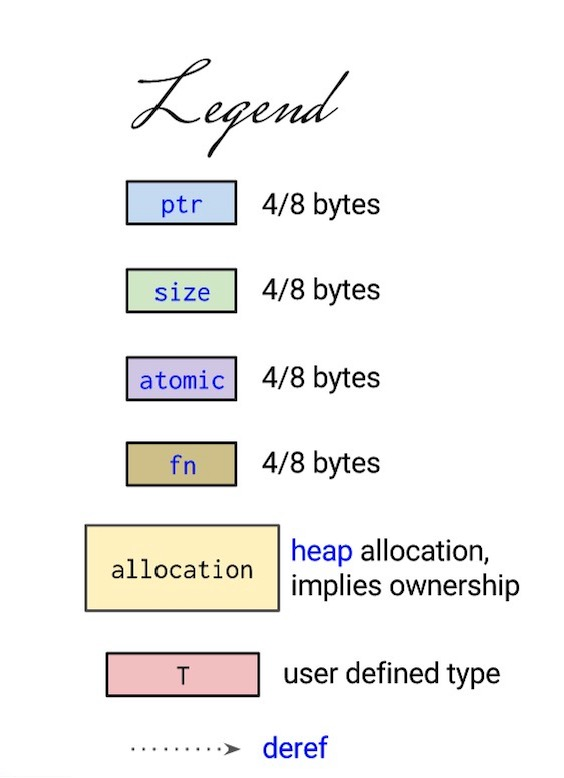Rust Language Cheat Sheet## GBK (GB2312/GB18030)

x00-xff GBK双字节编码范围
x20-x7f ASCII
xa1-xff 中文
x80-xff 中文


## UTF-8 (Unicode)

u4e00-u9fa5 (中文)
x3130-x318F (韩文)
xAC00-xD7A3 (韩文)
u0800-u4e00 (日文)
uff21 – uff5a 英文全角 A-z
uff01 - uff09 美式键盘 1-9 上标字符  02 双引号 06 中文省略号……
uff10 - uff19 全角数字  ０ – ９
uff20 @


9836266C
&#9836;&#x266C;

## 示例

$$x+\sqrt{1-x^2}$$


$$x+\sqrt{1-x^2}$$

$$y=\frac1{1-x^2}$$


$$y=\frac1{1-x^2}$$

... we have $x\_1 = 132$ and $x\_2 = 370$ and so ...


... we have $x_1 = 132$ and $x_2 = 370$ and so ...

## 1. 安装

1. 需要 java 环境。
2. 需要安装 [Graphviz] 依赖
3. 下载 plantuml

## 2. 使用命令

planuml.jar 同级别文件夹建立 planuml.bat. 类 Unix 系统中使用 bash 即 .sh 脚本，或者创建 alias 别名。

java -jar plantuml.jar -gui


window 下使用 ansi 编码保存puml文件(默认的 txt 文档，修改 pu 为后缀)。

## 1. 安装

cargo install mdbook


## 2. 使用

mdbook 命令

• help 查看帮助
• init 创建和初始化文档
• build 生成 book
• serve 建立本地服务，默认地址为 http://localhost:3000
• watch 监控文件变动